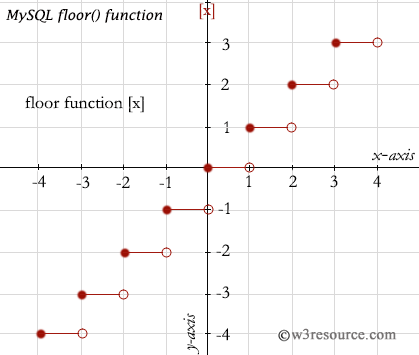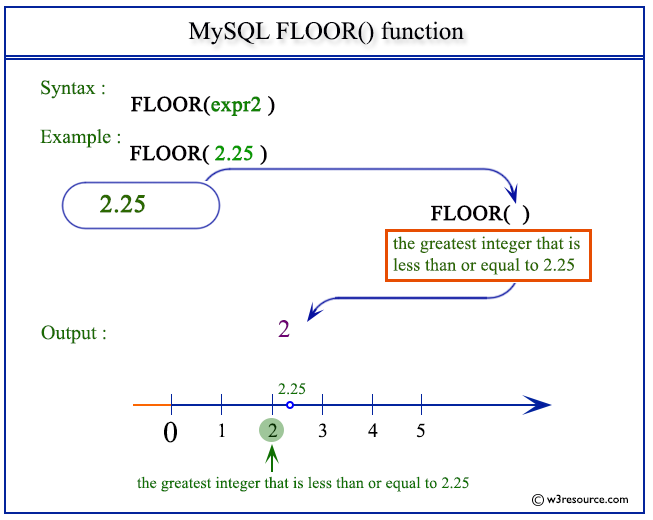# MySQL FLOOR() function

## FLOOR() function

MySQL FLOOR() returns the largest integer value not greater than a number specified as an argument.

Syntax:

```FLOOR(N);
```

Argument:

Name Description
N A number.

Syntax Diagram:MySQL Version: 5.6

Pictorial presentation of FLOOR() FunctionPictorial presentation of MySQL FLOOR() functionExample of MySQL FLOOR() function

The following MySQL statement returns 1 which is the largest integer value of given number (1.72) as specified in the argument.

Code:

``````SELECT FLOOR(1.72);
```
```

Sample Output:

```mysql> SELECT FLOOR(1.72);
+-------------+
| FLOOR(1.72) |
+-------------+
|           1 |
+-------------+
1 row in set (0.02 sec)
```

Example: FLOOR() with negative value

The following MySQL statement return -3 which is not the largest integer value of given number (-2.72) as specified in the argument.

Code:

``````SELECT FLOOR (-2.72);
```
```

Sample Output:

```mysql> SELECT FLOOR (-2.72);
+---------------+
| FLOOR (-2.72) |
+---------------+
|            -3 |
+---------------+
1 row in set (0.00 sec)
```

All Mathematical FunctionsPrevious: EXP()
Next: LN()

﻿